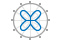# ezpolar

Easy-to-use polar coordinate plotter

•## Syntax

```ezpolar(fun) ezpolar(fun,[a,b]) ezpolar(axes_handle,...) h = ezpolar(...) ```

## Description

`ezpolar(fun)` plots the polar curve `rho = fun(theta)` over the default domain 0 < `theta` < 2π.

`fun` can be a function handle, a character vector, or a string (see the Tips section).

`ezpolar(fun,[a,b])` plots `fun` for `a` < `theta` < `b`.

`ezpolar(axes_handle,...)` plots into the axes with handle `axes_handle` instead of the current axes (`gca`).

`h = ezpolar(...)` returns the handle to a line object in `h`.

## Examples

collapse all

Plot the function $1+\mathrm{cos}\left(t\right)$ over the domain $\left[0,2\pi \right]$.

```figure ezpolar('1+cos(t)')```## Tips

### Passing the Function as a Character Vector or String

Array multiplication, division, and exponentiation are always implied in the expression you pass to `ezpolar`. For example, the MATLAB® syntax for a plot of the expression

`t.^2.*cos(t)`

which represents an implicitly defined function, is written as

`ezpolar('t^2*cos(t)')`

That is, `t^2` is interpreted as `t.^2` in the character vector or string you pass to `ezpolar`.

### Passing a Function Handle

Function handle arguments must point to functions that use MATLAB syntax. For example, the following statements define an anonymous function and pass the function handle `fh` to `ezpolar`.

```fh = @(t) t.^2.*cos(t); ezpolar(fh)```

Note that when using function handles, you must use the array power, array multiplication, and array division operators (`.^, .*, ./`) since `ezpolar` does not alter the syntax, as in the case with character vector or string inputs.

If your function has additional parameters, for example `k1` and `k2` in `myfun`:

```function s = myfun(t,k1,k2) s = sin(k1*t).*cos(k2*t);```

then you can use an anonymous function to specify the parameters:

`ezpolar(@(t)myfun(t,2,3))`

## Version History

Introduced before R2006a